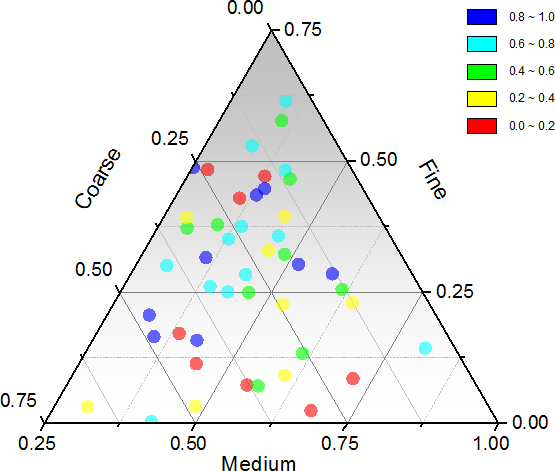Skip Navigation Links.Description:

This Ternary plot was created from a worksheet with four columns of data. The first three columns contained the ternary coordinates of the scatter points. In addition, the symbol color is mapped to the fourth column. The gradient filled background color is controlled on the Background tab of the Plot Details dialog.

Ternary plot assumes that the input coordinates are normalized, which means that the sum of the absolute values of cells in every row equals to 1 or 100: |x|+|y|+|z|=1 or 100. If the input data has at least one data point that is not normalized, a Reminder Message dialog box will be showed to ask you whether to normalize your data before the ternary plot is created.

Origin also allows you to rescale the ternary plot to a region of interest. XYZ axes are strict tied in the relationship that x+y+z=1.